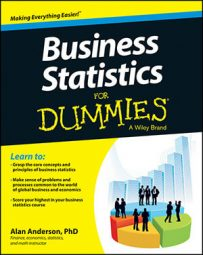Regression analysis is a statistical tool used for the investigation of relationships between variables. Usually, the investigator seeks to ascertain the causal effect of one variable upon another — the effect of a price increase upon demand, for example, or the effect of changes in the money supply upon the inflation rate.

Regression analysis is used to estimate the strength and the direction of the relationship between two linearly related variables: X and Y. X is the "independent" variable and Y is the "dependent" variable.

The two basic types of regression analysis are:

• Simple regression analysis: Used to estimate the relationship between a dependent variable and a single independent variable; for example, the relationship between crop yields and rainfall.

• Multiple regression analysis: Used to estimate the relationship between a dependent variable and two or more independent variables; for example, the relationship between the salaries of employees and their experience and education.

Multiple regression analysis introduces several additional complexities but may produce more realistic results than simple regression analysis.

Regression analysis is based on several strong assumptions about the variables that are being estimated. Several key tests are used to ensure that the results are valid, including hypothesis tests. These tests are used to ensure that the regression results are not simply due to random chance but indicate an actual relationship between two or more variables.

An estimated regression equation may be used for a wide variety of business applications, such as:

• Measuring the impact on a corporation's profits of an increase in profits

• Understanding how sensitive a corporation's sales are to changes in advertising expenditures

• Seeing how a stock price is affected by changes in interest rates

Regression analysis may also be used for forecasting purposes; for example, a regression equation may be used to forecast the future demand for a company's products.

Due to the extreme complexity of regression analysis, it is often implemented through the use of specialized calculators or spreadsheet programs.